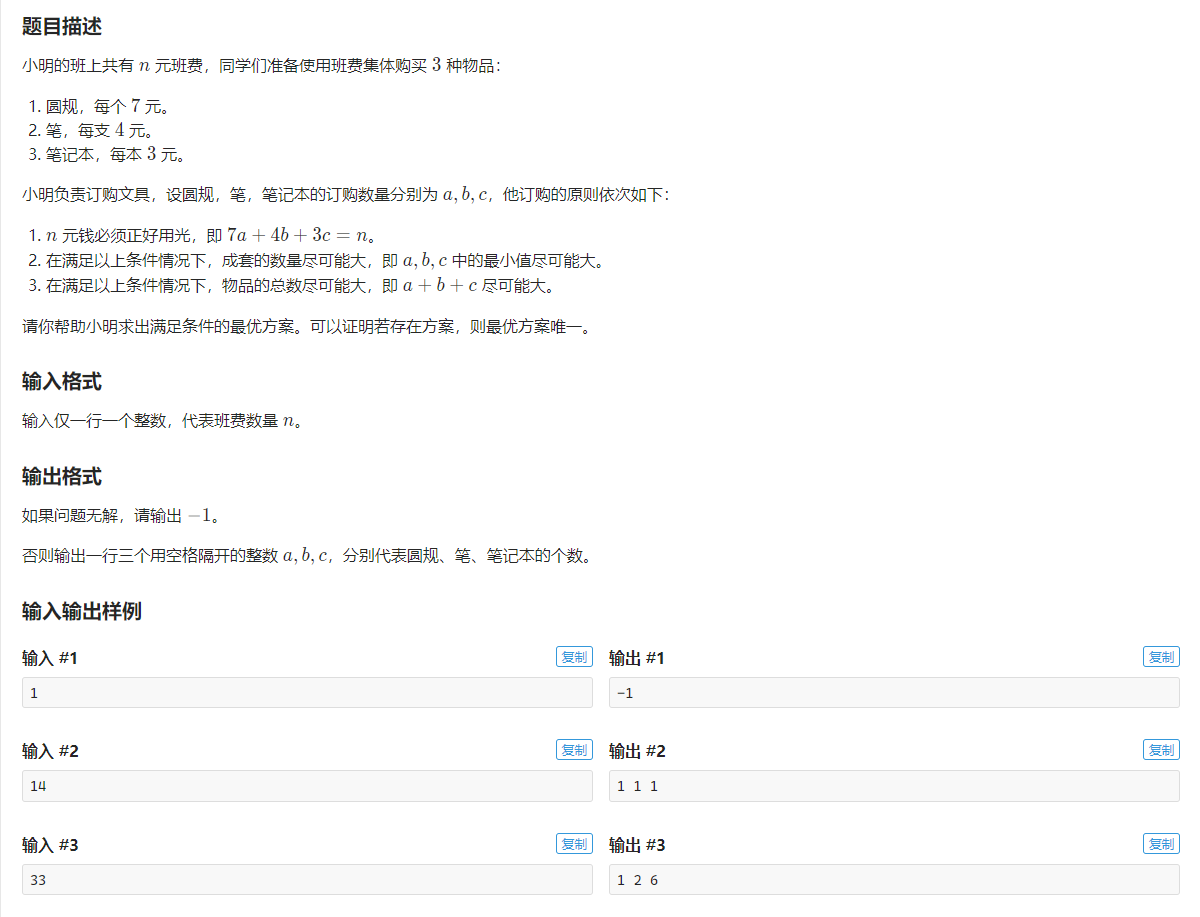# NOI Online 普及组 解题报告

## # T1• $$\because 7a+4b+3c=n$$
• $$\therefore 7a=n-4b-3c$$
• 由于我们要考虑 $a+b+c$ 最大. 因为 $b$ 的系数比 $c$ 要大, 而和为定值, 所以当 $a$ 固定时, $b$ 越小越好
• 所以我们就可以令 $b$ 为 $a, a+1, a+2$ 中的一个(为了满足 $c$ 是整数, 找一个值使得 $n-4b-7a \equiv 0 \pmod {3}$)